# PostgreSQL WHERE

## WHERE Clause

The PostgreSQL WHERE clause is used to control a PostgreSQL SELECT query, i.e. records or rows can be fetched according to an expression or some conditions supplied by the user.

In a PostgreSQL SELECT statement the FROM clause sends the rows into a consequent table temporarily, therefore each row of the resultant table is checked against the search condition. Rows which match the condition remains in the output table. The search condition required at least one column of the table specified after FROM clause.

Syntax:

`WHERE search_condition`

Parameter

Parameter Description
search_condition A value expression. It returns a value of type boolean.

Create Table

Code:

``````CREATE table actor(actor_id character(15), first_name character(15),last_name character(15),actor_age numeric(2), last_update time without time zone
```
```

Insert data

Code:

``````INSERT INTO actor values ('ACT001','Alan','Nixon',26,'15:22:43'); INSERT INTO actor values ('ACT002','Zeon','Haus',28,'07:15:14'); INSERT INTO actor values ('ACT003','Ramsekhar','Alfanso',26,'11:47:23'); INSERT INTO actor values ('ACT004','McKord','Hill',31,'09:36:45');
```
```

Table Structure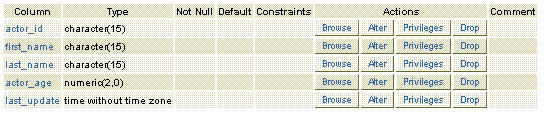Data available in the table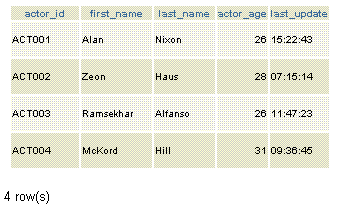PostgreSQL WHERE example1

If we want to fetch all rows from the actor table which satisfy the condition last_name is 'Haus' the following PostgreSQL SELECT statement can be used.

SQL

Code:

``````SELECT * FROM actorWHERE last_name='Haus'
```
```

Output: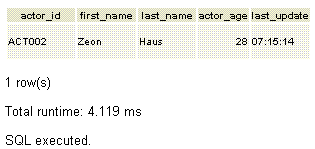PHP with PostgreSQL WHERE Clause example 1

Code:

``````<!DOCTYPE html>
<html lang="en">
<meta charset="utf-8">
<title>PostreSQL PHP WHERE Example 1</title>
<meta name="description" content="If we want to fetch all rows from the actor table which satisfy the condition last_name is 'Haus' the following PostgreSQL SELECT statement can beused.">
<body>
<h1>List of all actors whose last name is Haus</h1>
echo "<table>";while(\$row=pg_fetch_assoc(\$result)){echo "<tr>";
echo "<td align='center' width='200'>" . \$row['actor_id'] . "</td>";
echo "<td align='center' width='200'>" . \$row['first_name'] . "</td>";
echo "<td align='center' width='200'>" . \$row['last_name'] . "</td>";
echo "<td align='center' width='200'>" . \$row['actor_age'] . "</td>";
echo "<td align='center' width='200'>" . \$row['last_update'] . "</td>";
echo "</tr>";}
echo "</table>";
?>
</body>
</html>
```
```

PostgreSQL WHERE example2

If we want to fetch all rows from the actor table which satisfy the condition actor_age is 26 the following PostgreSQL SELECT statement can be used.

Code:

``````SELECT * FROM actor WHERE actor_age=26
```
```

Output: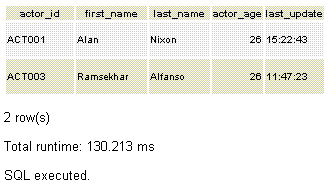PHP with PostgreSQL WHERE Clause example 2

Code:

``````<!DOCTYPE html>
<html lang="en">
<meta charset="utf-8">
<title>PostreSQL PHP WHERE Example 2</title>
<meta name="description" content="If we want to fetch all rows from the actor table which satisfy the condition actor_age is 26 the following PostgreSQL SELECT statement can be used.">
<body>
<h1>List of all actors those who are 26 </h1>
echo "<table>";while(\$row=pg_fetch_assoc(\$result)){echo "<tr>";
echo "<td align='center' width='200'>" . \$row['actor_id'] . "</td>";
echo "<td align='center' width='200'>" . \$row['first_name'] . "</td>";
echo "<td align='center' width='200'>" . \$row['last_name'] . "</td>";
echo "<td align='center' width='200'>" . \$row['actor_age'] . "</td>";
echo "<td align='center' width='200'>" . \$row['last_update'] . "</td>";
echo "</tr>";}echo "</table>";?>
</body>
</html>
```
```

PostgreSQL WHERE example3

If we want to fetch all rows from the actor table which satisfy the condition actor_age is more than 26 the following PostgreSQL SELECT statement can be used.

Code:

``````SELECT *FROM actor WHERE actor_age>26
```
```

Output: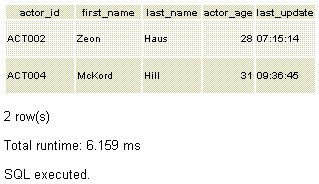PHP with PostgreSQL WHERE clause example 3

Code:

``````<!DOCTYPE html>
<html lang="en">
<meta charset="utf-8">
<title>PostreSQL PHP WHERE Example 2</title>
<meta name="description" content="If we want to fetch all rows from the actor table which satisfy the condition actor_age is 26 the following PostgreSQL SELECT statement can be used.">
<body>
<h1>List of all actors those who are older than 26 </h1>
echo "<table>";while(\$row=pg_fetch_assoc(\$result)){echo "<tr>";
echo "<td align='center' width='200'>" . \$row['actor_id'] . "</td>";
echo "<td align='center' width='200'>" . \$row['first_name'] . "</td>";
echo "<td align='center' width='200'>" . \$row['last_name'] . "</td>";
echo "<td align='center' width='200'>" . \$row['actor_age'] . "</td>";
echo "<td align='center' width='200'>" . \$row['last_update'] . "</td>";
echo "</tr>";}echo "</table>";?>
</body>
</html>
``````
``` ```

Previous: SELECT Statement
Next: DISTINCT Clause

﻿